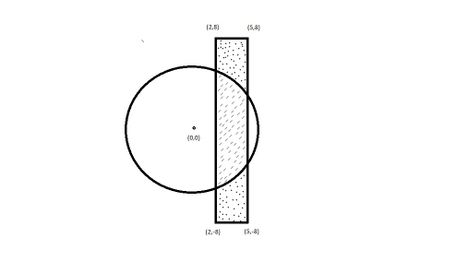# Infinite Gameflash_7The Tough Summer Spar 201...
Limits 1s, 512 MB

One day Oditi and her two brothers Sam and Ron were feeling bored as their summer vacation was almost getting over. Then suddenly they planned for an interesting game. There was an infinite grid land and the game was about having as much area of it as they can take. But there are some rules.

Oditi is at co-ordinate $(0, 0)$ and for a given R, she can only reach the land which are not more than R distance from her own position. Sam is at position $(x1, -y)$ and Ron is at $(x2, -y)$. Sam wants to reach at $(x1, y)$ and Ron wants to reach at $(x2, y)$. So Sam will start running from $(x1, -y)$ and Ron will start running from $(x2, -y)$ through a straight line until they reach their destination. They will be able to reach the land that lie between their path.

But they also have another little cousin named Tom. Tom will not play the game but he also wants to get some part of the land. Otherwise he won’t let them play.

As Oditi is the eldest, she made the final decision. Tom will get the area which is reachable by both Oditi and her brothers (Sam and Ron). And excluding the area that Tom gets, Oditi will get the rest of the area which are reachable from her. Sam and Ron will both get half of the area that are reachable from them excluding the area Tom gets.

Now you have to calculate the area which will be taken by Oditi, Sam, Ron and Tom.

The picture illustrates the first case of sample input. Here Tom gets the intersected lined area, Sam and Ron both gets the half of the dotted area. And Oditi gets the blank area inside the circle.## Input

The first line contains an integer number $T$ ($1 ≤ T ≤ 10^3$) which is the number of test cases. For each test case, there will be a single line which contains 4 decimal numbers $R$, $x1$, $x2$ ($1 ≤ x1, x2, R ≤ 10^3$) and $y$ ($R ≤ y ≤ 10^3$). $y$ is always greater than $R$ and $x1 \ne x2$.

## Output

For each test case, print four space separated values, the area of the lands Oditi, Sam, Ron and Tom will get. Always print 3 digits after the decimal point.

## Sample

InputOutput
4
6 2 5 8
6 2 5 10
10 6 11 15
5 3 5 8

84.598 9.750 9.750 28.499
84.598 15.750 15.750 28.499
269.430 52.635 52.635 44.730
67.357 10.409 10.409 11.182GeometryuDebug

### Submit